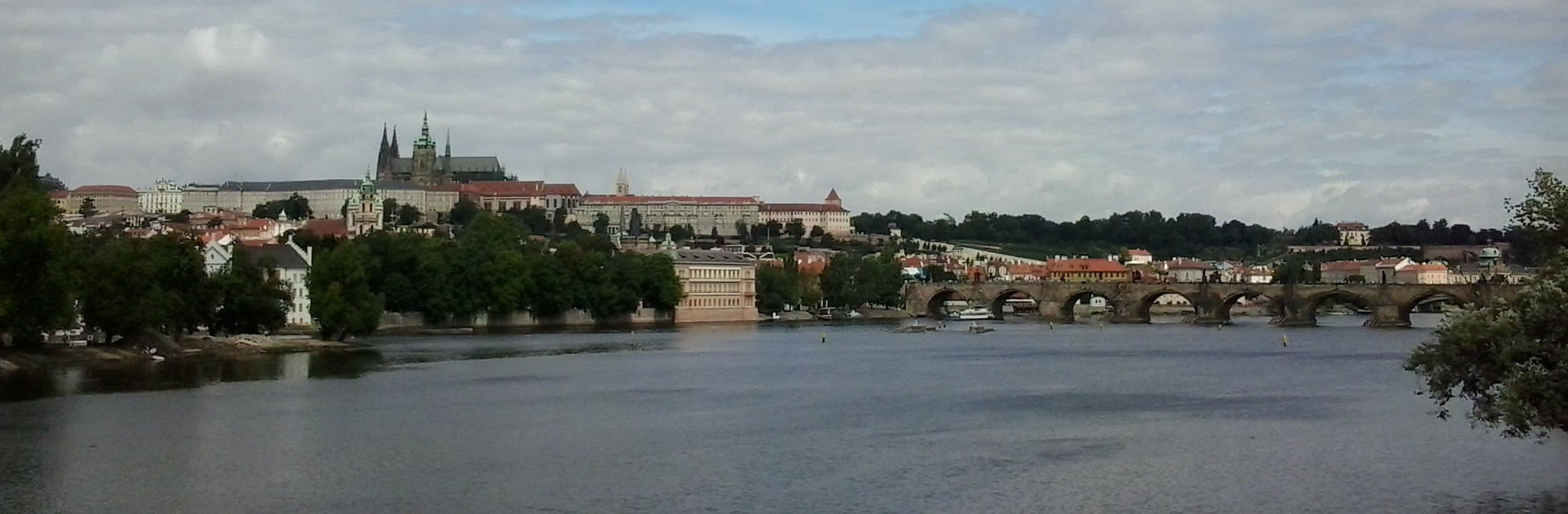### Research interests

Most of my work belongs to discrete mathematics. This area of mathematics deals with mathematical structures and problems that are "discrete" in nature, as opposed to those that exhibit continuous properties. Since information in computer systems is stored as sequences of zeroes and ones, discrete mathematics finds many applications in computer science.

My PhD is in structural and algorithmic graph theory. A graph is a mathematical object that consists of elements called vertices and links between them called edges. For example, the road map in a GPS device in a car is discretized and represented by a graph, i.e. by a list of crossings (vertices) with mutual links (edges). A substantial amount of my work during and after my PhD is related to graph colorings, i.e. decompositions of vertices and edges of graphs subject to certain constraints. In relation to computer science applications, I am interested in using graph decompositions and logic methods in algorithm design.

Most of my current research is related to combinatorial limits. The theory of combinatorial limits provides analytic views on large discrete structures and it responds to challenges from computer science where structures such as the graph of internet connections and graphs of social networks (e.g., Facebook, LinkedIn) are enormous. The theory opened new links between analysis, combinatorics, ergodic theory, group theory and probability theory. For example, one of the major problems on sparse graph limits, the conjecture of Aldous and Lyons, is essentially equivalent to Gromov's question whether all countable discrete groups are sofic. My work includes applications of analytic methods in extremal combinatorics and it also led to new insights in structural properties of graph limits.

### Highlights from recent work

#### Graph limits

Concerning the theory of graph limits, I have been mostly interested in problems concerning limits of dense graphs. Such graph limits determined by finitely many subgraph densities (finitely forcible graph limits) form extremal points of the graph limit space and are closely related to problems in extremal combinatorics, one of my main research areas in mathematics. Indeed, questions from extremal graph theory can be cast as optimization problems over the graph limit space with optimal solutions corresponding to its extremal points. It was believed that a special structure of finitely forcible graph limits could result in new methods for difficult problems from extremal combinatorics.

Lovász and Szegedy in 2011 made two conjectures that would imply that all finitely forcible graph limits possess a simple structure. In a series of papers coauthored by my postdocs and students, we developed the method of decorated constraints that can be used to construct complex finitely forcible graph limits, and disproved the two conjectures of Lovász and Szegedy. This line of research culminated with a joint result with Cooper and Martins (two students working under my supervision), where we provided a universal framework for constructing very complex finitely forcible graph limits by showing that any graphon can be embedded in a finitely forcible graphon (graphons are analytic objects representing dense graph limits). This dismissed any hope that finitely forcible graph limits possess a simple structure, and is surprising when contrasted with the fact that finitely forcible graph limits form a meager set in the space of all graph limits.

Building on these results, Grzesik, Lovász Jr. and I constructed a counterexample to a conjecture of Lovász on the finite forcibility of optimum solutions in extremal graph theory, which was one of the two most frequently cited open problems on graph limits. This conjecture, which is often referred to as saying that "every extremal graph theory problem has a finitely forcible optimum", reads as follows: every finite feasible set of subgraph density constraints can be extended further by a finite set of density constraints such that the resulting set is satisfied by an asymptotically unique graph. Such a result would match our empirical experience in extremal graph theory where many extremal configurations have a simple (though sometimes recursive) structure. We have constructed a counterexample using a mixture of analytic and flag algebra arguments.

• J. W. Cooper, D. Kráľ, T. Martins: Finitely forcible graph limits are universal, Advances in Mathematics 340 (2018), 819-854. Preprint available as arXiv:1701.03846.
• A. Grzesik, D. Kráľ, L. M. Lovász: Elusive extremal graphs, Proceedings of the LMS 121 (2020), 1685-1736. Preprint available as arXiv:1807.01141.

#### Graph coloring

Graph coloring is one of the most classical topics in graph theory and it continues to form a large part of my research since my PhD. A major open problem on colorings of planar graphs was Steinberg's Conjecture from 1976, which was widely believed to be true but out of reach of the current proof techniques. The conjecture asserts that every planar graph with no cycles of length four or five is 3-colorable and can be viewed as a variant of the very classical theorem of Grötzsch from 1959 that every triangle-free planar graph is 3-colorable. Steinberg's Conjecture has been attracting a substantial amount of attention among graph theorists and several stronger conjectures, e.g., Novosibirsk 3-Color Conjecture and Strong Bordeaux Conjecture, have been proposed. We disproved the conjecture in 2016. Steinberg's Conjecture is the first and, so far, the only solved problem with a four star (highest) importance rating on the Open Problem Garden, a collection of open problems particularly from combinatorics. Our (quite simple) counterexample attracted a good amount of attention in the graph theory community and was also featured in an article in the popular science magazine Pour la Science.

• V. Cohen-Addad, M. Hebdige, D. Kráľ, Z. Li, E. Salgado: Steinberg's Conjecture is false, Journal of Combinatorial Theory Ser. B 122 (2017), 452-456. Preprint available as arXiv:1604.05108.